微信聊天记录数据分析

工作内容

• 获取微信聊天数据库
• 解密数据库获取聊天数据
• 使用Python分析数据
• ECharts(或者pyecharts)做数据可视化

获取聊天数据库

• 首先需要获取手机root权限

• 安装RE文件管理器(Root Explorer)

• 打开RE文件管理器，在路径/data/data/com.tencent.mm/MicroMsg下找到一个由32位字符串命名的文件夹，比如我的文件夹如下，这个32位字符串是mm+uin码进行md5加密后得到的(uin码后面会说)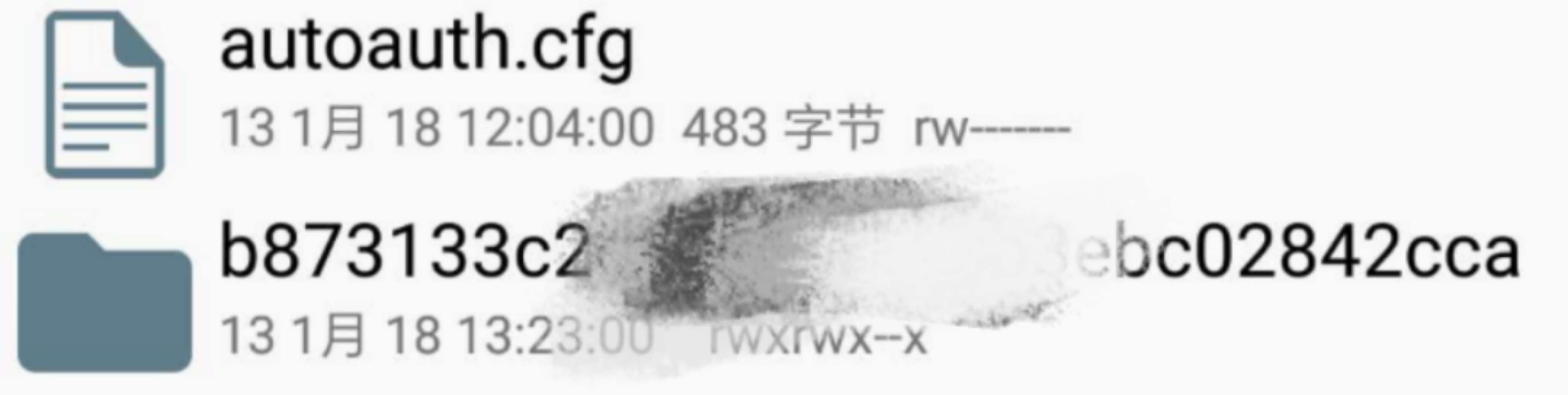• 进入该文件夹，找到EnMicroMsg.db，即为你的微信聊天数据库，将其复制出来并传到电脑上

解密数据库获取聊天数据

• 获取IMEI码，即打开手机的拨号界面，输入* #06#，这时就会自动弹出你的IMEI码，但是对于双卡用户，操作的时候会有些懵，比如我用的小米6，输入* #06#后会出来3个码……其实也不用懵，MEID码直接无视，下面两个IMEI码的话，一般都是取第一个，当然不放心的话可以两个都试试。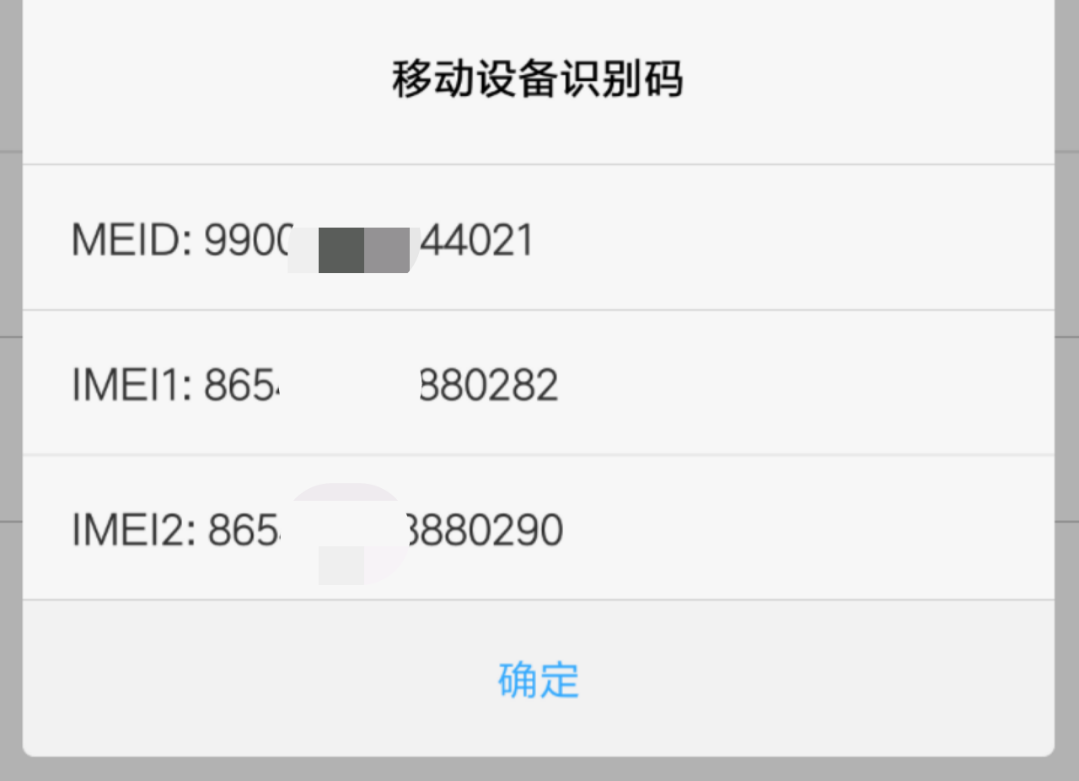• 获取uin码，再次打开RE文件管理器，在路径/data/data/com.tencent.mm/shared_prefs下找到auth_infokey_prefs.xml文件并打开，找到如下文字，其中value后面跟着的就是你的uin码了，我的是8位，每个人uin码的位数可能不一样。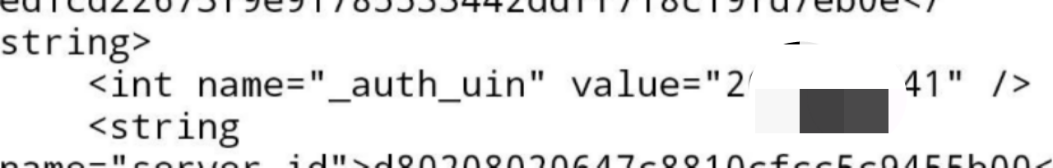• 在获取到IMEI码和uin码之后就可以相加后使用md5加密得到密码了。加密可以使用在线md5工具，密码是小写32位md5值的前7位。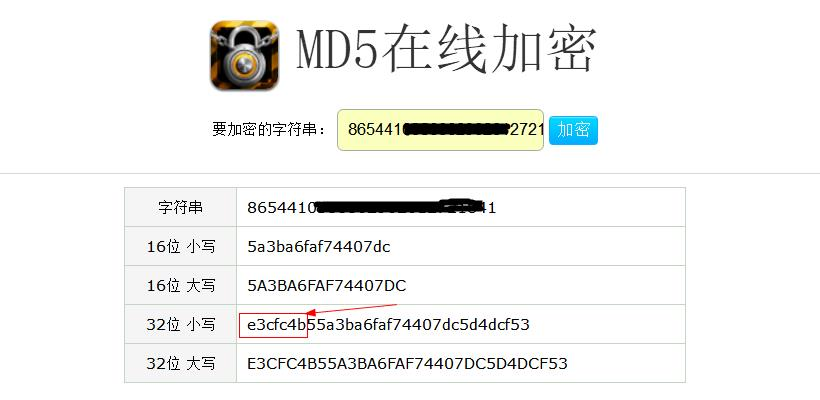• 获取到密码后就是解密数据库了。工具是sqlcipher.exe，我在网上找了很多版本，这个是最好用的：https://pan.baidu.com/s/1pLlLOA6ZDV5Nl0beMFvM4A （密码：kfe2）。打开后找到message表，这里就是全部的聊天记录了，关键的信息就是createTime、talker以及content这三列了，值得注意的是，数据库中保存的时间数据是时间戳，并且每一个数据后面都多出了三位0，在进行数据分析时需要把这三个0剔除。然后我们依次点击File–>Export–>Table as CSV file，将message表导出，我们就可以开始进行数据分析了。导出的文件默认有.csv和.txt两种，后缀需要手动写上去。此外，如果直接读取这个文件的话可能会存在编码错误的问题，建议在保存csv文件以后，右键使用记事本打开，然后再另存为一个新的文件，编码使用utf-8。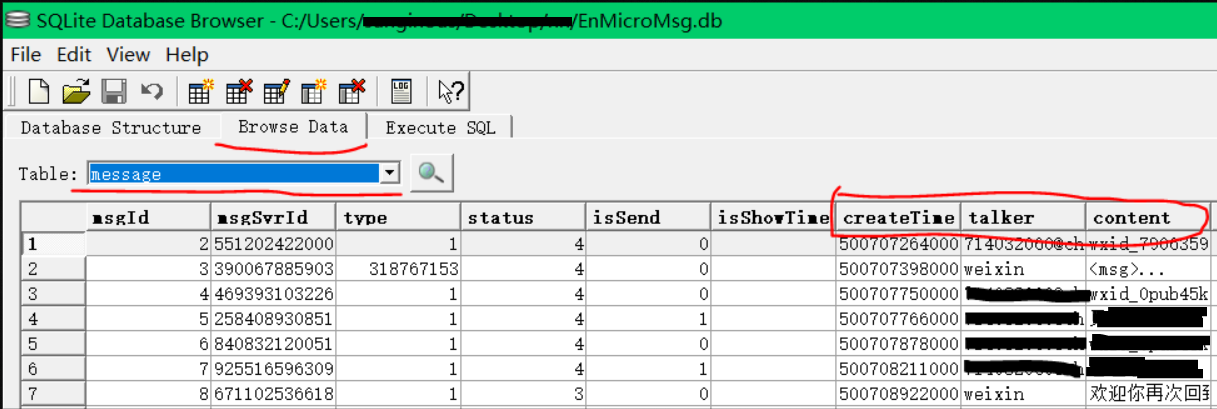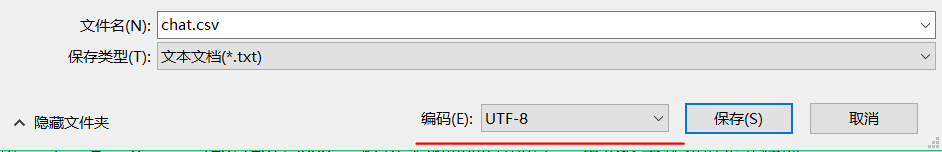使用Python分析数据

读取数据

chat = pd.read_csv('chat.csv', sep=',', usecols=[6,7,8])


myGirl = 'wxid_xxxxxxxx'
chat_time = []
chat_content = []
for i in range(len(chat)-1):
content = chat[i:i+1]
if content['talker'].values == myGirl:
t = content['createTime'].values//1000#除以1000用以剔除后三位0
c = content['content'].values
chat_time.append(t)
chat_content.append(c)


def to_hour(t):
struct_time = time.localtime(t)#将时间戳转换为struct_time元组
hour = round((struct_time + struct_time / 60), 2)
return hour

hour_set = [to_hour(i) for i in chat_time]

绘图

import seaborn as sns
from matplotlib.font_manager import *#如果想在图上显示中文，需导入这个包
myfont = FontProperties(fname=r'C:\Windows\Fonts\MSYH.TTC',size=22)#标题字体样式
myfont2 = FontProperties(fname=r'C:\Windows\Fonts\MSYH.TTC',size=18)#横纵坐标字体样式
sns.set_style('darkgrid')#设置图片为深色背景且有网格线
sns.distplot(hour_set, 24, color='lightcoral')
plt.xticks(np.arange(0, 25, 1.0), fontsize=15)
plt.yticks(fontsize=15)
plt.title('聊天时间分布', fontproperties=myfont)
plt.xlabel('时间段', fontproperties=myfont2)
plt.ylabel('聊天时间分布', fontproperties=myfont2)
fig = plt.gcf()
fig.set_size_inches(15,8)
fig.savefig('chat_time.png',dpi=100)
plt.show()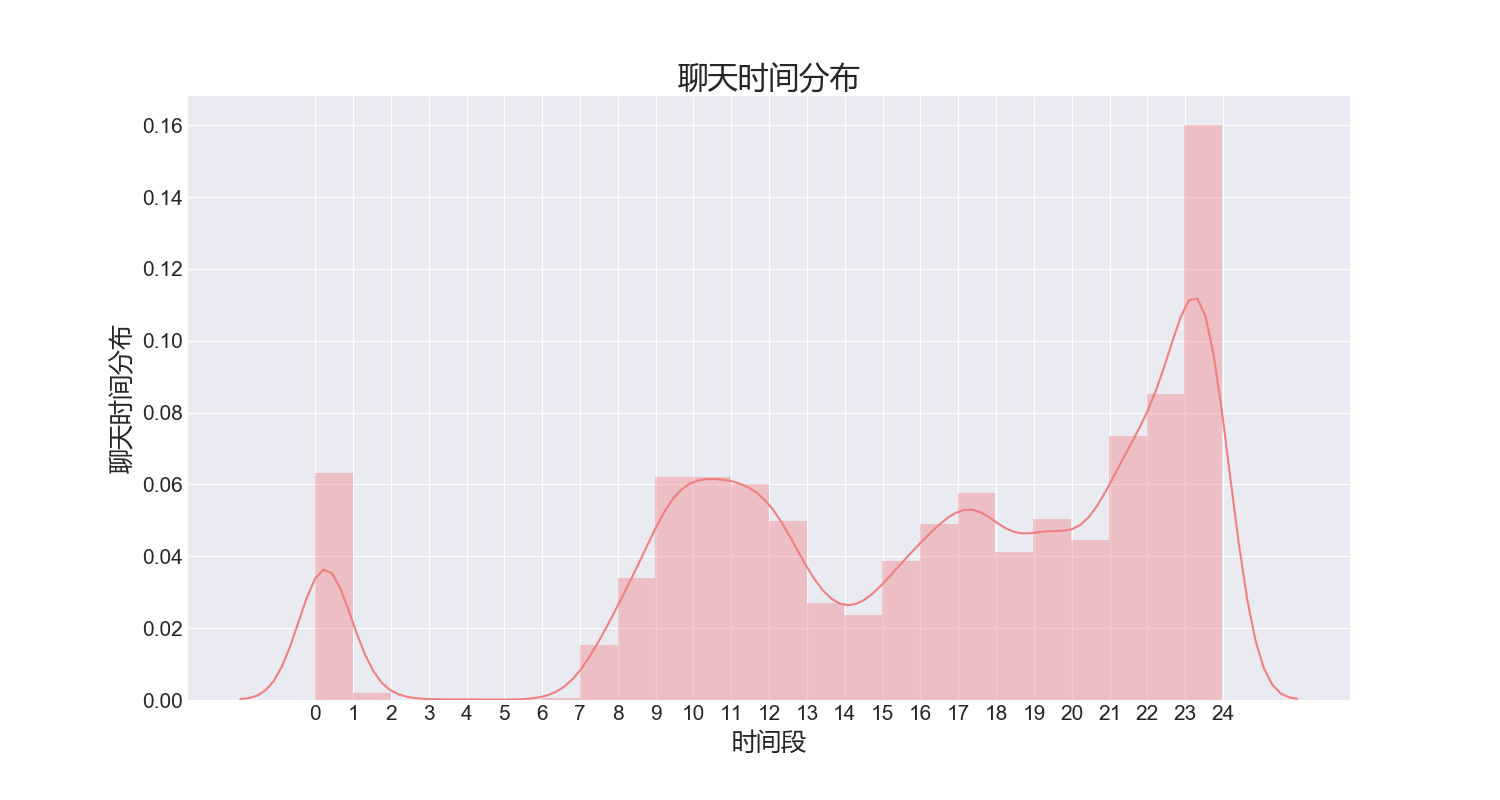使用ECharts

ECharts(http://echarts.baidu.com/)是一个开源的js组件，绘制的图表种类繁多而且很好看，使用起来也很方便，当然如果你并没有接触过js甚至对其有些抵触，那么也可以安装pyecharts(https://github.com/pyecharts/pyecharts)，可以看做是ECharts的Python版，操作更为简洁。这里我使用的是ECharts。首先我们需要在Python中统计hour_set里不同时间段的聊天记录条数，时间段可以任意划分，然后打开ECharts官网，找到官方实例中的雷达图，将统计出的各时间段聊天数据直接替换进去就可以了：

option = {
title: {
text: '聊天时间段',
textStyle: {
color: '#000',
fontSize: 20
}
},
//toolbox配置项可以在网页上直接生成下载图片的按钮而不用截图
toolbox: {
show: true,
feature: {
saveAsImage: {
show:true,
excludeComponents :['toolbox'],
pixelRatio: 2
}
}
},
tooltip: {},
// shape: 'circle',
name: {
textStyle: {
color: '#fff',
backgroundColor: '#999',
}
},
indicator: [
{ name: '凌晨2点至6点', max: 400},
{ name: '6点至10点次', max: 400},
{ name: '10点至14点', max: 400},
{ name: '14点至18点', max: 400},
{ name: '18点至22点', max: 400},
{ name: '22点至次日凌晨2点', max: 400}
]
},
series: [{
name: '聊天时间段',
// areaStyle: {normal: {}},
data : [
{
value : [63, 141, 250, 213, 263, 390],
name : '聊天时段'
}
]
}]
};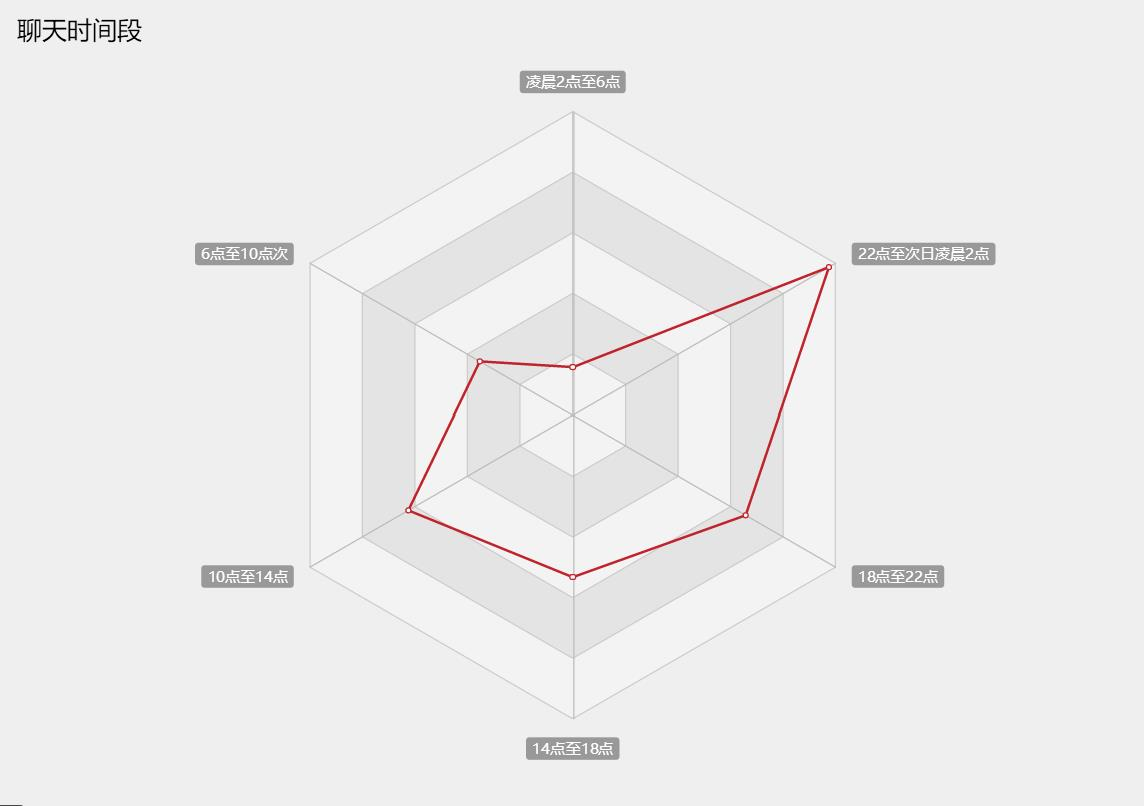统计特殊数据绘制字符云

import xlwt
wbk = xlwt.Workbook()
for i in range(len(deep_night)):
sheet.write(i,0,time.strftime('%Y-%m-%d %H:%M:%S',time.localtime(deep_night[i])))
sheet.write(i,1,deep_night[i])
wbk.save('聊得很晚.xls')


option = {
tooltip: {},
series: [{
type: 'wordCloud',
gridSize: 20,
sizeRange: [12, 50],
rotationRange: [0, 45],
shape: 'circle',
textStyle: {
normal: {
color: function() {
return 'rgb(' + [
Math.round(Math.random() * 200),
Math.round(Math.random() * 200),
Math.round(Math.random() * 200)
].join(',') + ')';
}
},
emphasis: {
}
},
data: [{
name: '呵呵',
value: 800,
textStyle: {
normal: {
color: 'black'
},
emphasis: {
color: 'red'
}
}
}, {
name: '别傻了，这是假数据',
value: 618
}, {
name: 'ECharts好用',
value: 438
}, {
name: '哈哈。。',
value: 405
}, {
name: '点个赞',
value: 246
}, {
name: '世界人民大团结万岁',
value: 224
}, {
name: 'naive',
value: 189
}, {
name: '还得学习一个',
value: 148
}, {
name: '闷声发大财！',
value: 111
}, {
name: '学习使我快乐',
value: 965
},  {
name: '葡萄牙1:0！！！',
value: 582
}, {
name: '+1s',
value: 555
}, {
name: '两点半的夜晚  哈哈哈哈',
value: 550
}]
}]
};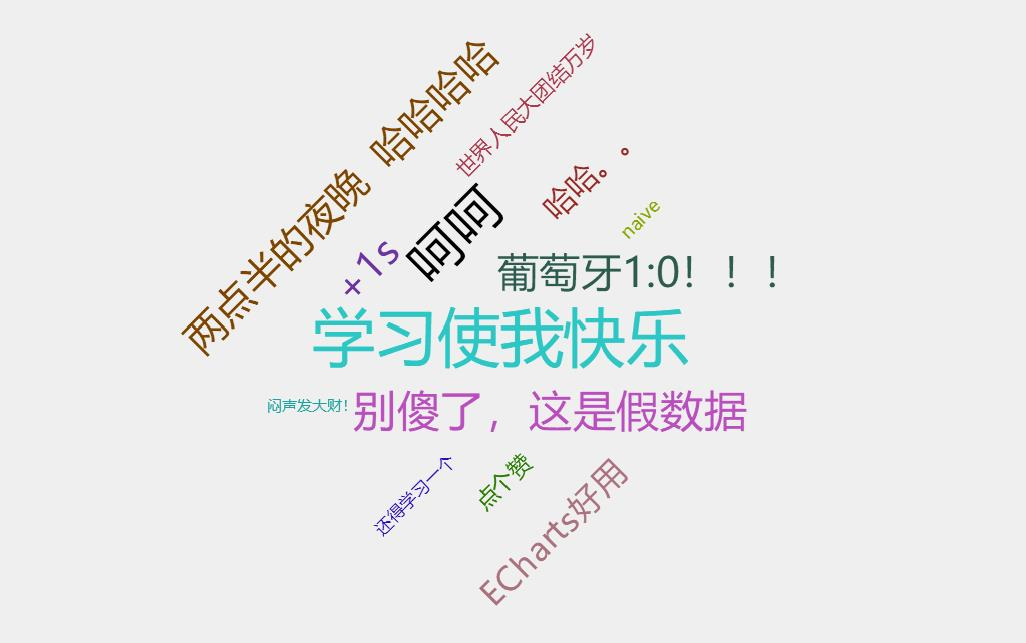匹配高频字符

import re
pattern = '.*?(爱).*?'
string = '我爱吃西瓜，你爱不爱吃。'
result = re.findall(pattern, string)
result
Out: ['爱', '爱', '爱']
len(result)
Out: 3


pattern_love = '.*?(爱).*?'
pattern_morning= '.*?(早安).*?'
pattern_night = '.*?(晚安).*?'
pattern_miss = '.*?(想你).*?'
pattern_set = [pattern_love, pattern_morning, pattern_night, pattern_miss]


start = datetime.datetime.now()
statistic = [0,0,0,0]
for i in range(len(chat_content)):
for j in range(len(pattern_set)):
length = len(re.findall(pattern_set[j], str(chat_content[i])))
static[j] += length
result = {
'爱': static,
'早安': static,
'晚安': static,
'想你': static
}
print(result)
end = datetime.datetime.now()
print('\n..........\n字符统计结束,用时: {}\n............\n'.format(end-start))


这一年

11月11日大概是很特别的一天

……


from operator import itemgetter
chat_all = []
myGirl = 'wxid_xxxxxxxx'
for i in range(len(chat)-1):
content = chat[i:i+1]
if content['talker'].values == myGirl:
t = content['createTime'].values//1000
c = content['content'].values
chat_all.append([t,c])
chat_all = sorted(chat_all, key=itemgetter(0))#以第一维为索引排序


import pandas as pd
import time
import seaborn as sns
import matplotlib.pyplot as plt
import datetime
import numpy as np
import re
from operator import itemgetter
from matplotlib.font_manager import *#导入这个包，可以添加中文字体
import xlwt

chat_file = '自己的csv文件'
myGirl = '目标联系人的微信号'

''' 读取原数据 '''
chat_time = []
chat_content = []
chat_all = []
for i in range(len(chat)-1):
content = chat[i:i+1]
if content['talker'].values == myGirl:
t = content['createTime'].values//1000
c = content['content'].values
chat_time.append(t)
chat_content.append(c)
chat_all.append([t,c])
chat_all = sorted(chat_all, key=itemgetter(0))#以第一维为索引排序

''' 转换时间格式 '''
def to_hour(t):
struct_time = time.localtime(t)
hour = round((struct_time + struct_time / 60), 2)
return hour
hour_set = [to_hour(i) for i in chat_time]

print('\n.......................\n开始画图\n.......................')
from matplotlib.font_manager import *#如果想在图上显示中文，需导入这个包
myfont = FontProperties(fname=r'C:\Windows\Fonts\MSYH.TTC',size=22)#标题字体样式
myfont2 = FontProperties(fname=r'C:\Windows\Fonts\MSYH.TTC',size=18)#横纵坐标字体样式
sns.set_style('darkgrid')#设置图片为深色背景且有网格线
sns.distplot(hour_set, 24, color='lightcoral')
plt.xticks(np.arange(0, 25, 1.0), fontsize=15)
plt.yticks(fontsize=15)
plt.title('聊天时间分布', fontproperties=myfont)
plt.xlabel('时间段', fontproperties=myfont2)
plt.ylabel('聊天时间分布', fontproperties=myfont2)
fig = plt.gcf()
fig.set_size_inches(15,8)
fig.savefig('chat_time.png',dpi=100)
plt.show()
print('\n.......................\n画图结束\n.......................')

''' 聊天时段分布 '''
print('\n.......................\n开始聊天时段统计\n.......................')
time_slice = [0,0,0,0,0,0]
deep_night = []
for i in range(len(hour_set)):
if hour_set[i]>=2 and hour_set[i]<6:
time_slice += 1
deep_night.append([chat_time[i], chat_content[i]])
elif hour_set[i]>=6 and hour_set[i]<10:
time_slice += 1
elif hour_set[i]>=10 and hour_set[i]<14:
time_slice += 1
elif hour_set[i]>=14 and hour_set[i]<18:
time_slice += 1
elif hour_set[i]>=18 and hour_set[i]<22:
time_slice += 1
else:
time_slice += 1
labels = ['凌晨2点至6点','6点至10点','10点至14点',
'14点至18点','18点至22点','22点至次日凌晨2点']
time_distribution = {
labels: time_slice,
labels: time_slice,
labels: time_slice,
labels: time_slice,
labels: time_slice,
labels: time_slice
}
print(time_distribution)

''' 深夜聊天记录 '''
wbk = xlwt.Workbook()
for i in range(len(deep_night)):
sheet.write(i,0,time.strftime('%Y-%m-%d %H:%M:%S',time.localtime(deep_night[i])))
sheet.write(i,1,deep_night[i])
wbk.save('聊得很晚.xls')
print('\n.......................\n聊天时段统计结束\n.......................')

''' 字符统计 '''
print('\n..........\n开始字符统计\n............\n')
start = datetime.datetime.now()
pattern_love = '.*?(爱).*?'
pattern_morning = '.*?(早安).*?'
pattern_night = '.*?(晚安).*?'
pattern_miss = '.*?(想你).*?'
pattern_set = [pattern_love, pattern_morning, pattern_night, pattern_miss]
statistic = [0,0,0,0]
for i in range(len(chat_content)):
for j in range(len(pattern_set)):
length = len(re.findall(pattern_set[j], str(chat_content[i])))
statistic[j] += length
result = {
'爱': statistic,
'早安': statistic,
'晚安': statistic,
'想你': statistic
}
print(result)
end = datetime.datetime.now()
print('\n..........\n字符统计结束,用时: {}\n............\n'.format(end-start))